# Quantum sort : sorting algorithm IBM Qiskit tutorial python

In this article, I propose a quantum sort algorithm with Qiskit tutorial in python that allows you to sort a list of integers coded on two qubits. I have found that there is little literature on the subject. This algorithm can be used and can be used as a basis for solving sorting problems on an unsorted list of integers.

## Introduction

We consider the following problem: Given a list of four integers between 0 and 3 that are strictly different sort the given list. The solution proposed is a quantum sort algorithm.

A classic sorting algorithm such as merge sorting solves the problem, see in more detail my article on the subject:

Merge sorting follows the divide and conquer paradigm of dividing the initial task into two similar smaller tasks.

## Some concepts of quantum algorithm and IBM Qiskit tutorial

I recall here some concept that we are going to use in our quantum sorting algorithm, so I advise you to consult the IBM Qiskit site to learn more https://qiskit.org/textbook/what-is-quantum.html and also to read the very good book “Programming Quantum Computers: Essential Algorithms and Code Samples” by Eric R. Johnston, Nic Harrigan, Mercedes Gimeno-Segovia:

###### Circuits

The principle in quantum algorithm is to manipulate qubits for inputs which we have in outputs which we need, the process can be represented in the form of circuit (inputs on the left, output on the right). We can perform different operations by means of quantum gate (which act on the qubits in a way similar in classical algorithm to logic gates like AND, OR, Exclusive OR…).

In this article I will rely on Qiskit which is the IBM framework for quantum computing.

from qiskit import QuantumRegister, ClassicalRegister, QuantumCircuit
from numpy import pi

qreg_qubit = QuantumRegister(2, 'qubit')
creg_classic = ClassicalRegister(2, 'classic')
circuit = QuantumCircuit(qreg_qubit, creg_classic)

circuit.reset(qreg_qubit)
circuit.reset(qreg_qubit)
circuit.x(qreg_qubit)
circuit.measure(qreg_qubit, creg_classic)
circuit.measure(qreg_qubit, creg_classic)

In this first algorithm, a quantum register is initialized to the state | 01> measures in a classical register the quantum state of a register of 2 qubits. The circuit can be drawn using the circuit.draw () instruction or via Circuit Composer from IBM Quantum Experience: https://quantum-computing.ibm.com/

###### Quantum state of a single qubit:

The state of a qubit can be expressed as a state vector: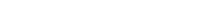With alpha and beta which are complex numbers (ie a real and imaginary part) in this case there is the relation (mathematical reminder on complex numbers: https://www.mathsisfun.com/numbers/complex-numbers.html):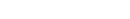If we go into trigonometric and exponential form we have with θ and ϕ which are real numbers.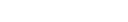So if we measure a qubit q we have: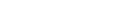That is, there is a probability of measuring the qubit in state | 0> and a probability of measuring the qubit in state | 1>. The measurement of a qubit reveals whether it is in state 1 or in state 0, in general the measurement is placed at the end of the circuit because it affects the state of the qubit accordingly.

###### Quantum gates

Here are some quantum gates to know that we will use in our quantum sorting algorithm:

Gate X (Pauli-X) :

Gate X is used to return the state of the qubit without modifying the phase: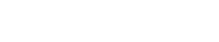See the example above with the use of qiskit.

Gate Y (Pauli-Y) :

The pauli Y gate allows you to return the phase and the qubit at the same time:

Example if we consider here the initialized state | 0> of the qubit and its phase of 0, there is then a 100% chance of measuring the state | 0>.

Applying the quantum gate Y has the following effect on the state and phase of the qubit:

There is then a 100% chance of measuring state 1 and the phase is reversed (see the bloch sphere above)

from qiskit import QuantumRegister, ClassicalRegister, QuantumCircuit
from numpy import pi

qreg_qubit = QuantumRegister(1, 'qubit')
creg_classic = ClassicalRegister(1, 'classic')
circuit = QuantumCircuit(qreg_qubit, creg_classic)

circuit.reset(qreg_qubit)
circuit.y(qreg_qubit)
circuit.measure(qreg_qubit, creg_classic)

Gate Z (Pauli-Z) :

The Z gate is used to return only the phase and not the state of the qubit.

Note that the Y and Z gates can be represented by the following matrices: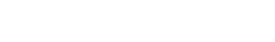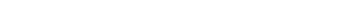The Hadamard gate (H gate) is an important quantum gate which allows to create a superposition of | 0⟩ and | 1⟩.

The matrix of this gate is: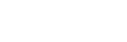SWAP Gate

A SWAP gate allows the state of two qubits to be exchanged:

SWAP state example | 1⟩ from qubit 0 to qbit 2

And state SWAP | 0⟩ from qubit 1 to qbit 2

In Python with the Qiskit framework we have:

from qiskit import QuantumRegister, ClassicalRegister, QuantumCircuit
from numpy import pi

qreg_qubit = QuantumRegister(3, 'qubit')
creg_classic = ClassicalRegister(1, 'classic')
circuit = QuantumCircuit(qreg_qubit, creg_classic)

circuit.reset(qreg_qubit)
circuit.reset(qreg_qubit)
circuit.reset(qreg_qubit)
circuit.x(qreg_qubit)
circuit.swap(qreg_qubit, qreg_qubit)
circuit.measure(qreg_qubit, creg_classic)

Toffoli Gate

The Toffoli quantum gate is three qubits (two commands and one target).
It performs a phase reversal (quantum gate X on the target) only if both controls are in state | 1⟩.
A Toffoli is equivalent to an UNControlled gate (it is also called the CCX gate).

Example below with 2 command qubits in state | 1⟩ there is then a 100% chance of measuring the state | 1⟩ on the target qubit of the tofoli gate which was initially initialized to state | 0⟩ (or a reversal of the state).

Example below in the opposite case (there is then 0% chance of measuring state | 1⟩ or 100% chance of measuring state | 0⟩):

###### Classical gate AND gate, OR gate equivalence in quantum gate – IBM Qiskit tutorial

We see here how to combine doors to create a behavior equivalent to what we are used to using in classical circuits.

Gate AND :

From Qbit a and b and a qubit with a forced initialization to state | 0⟩ we can obtain the operation a AND b using a Toffoli quantum gate and a SWAP mate.Le circuit suivant effectue un classique ET sur un algorithme quantique

It should be noted that at the end of this circuit we find the state A AND B on qubit B the state of qubit B is ultimately on qubit C.

The equivalent python code is as follows:

from qiskit import QuantumRegister, ClassicalRegister, QuantumCircuit
from numpy import pi

qreg_a = QuantumRegister(1, 'a')
qreg_b = QuantumRegister(1, 'b')
qreg_c = QuantumRegister(1, 'c')
creg_aANDb = ClassicalRegister(1, 'aANDb')
creg_aState = ClassicalRegister(1, 'aState')
creg_bState = ClassicalRegister(1, 'bState')
circuit = QuantumCircuit(qreg_a, qreg_b, qreg_c, creg_aANDb, creg_aState, creg_bState)

circuit.reset(qreg_c)
circuit.ccx(qreg_a, qreg_b, qreg_c)
circuit.swap(qreg_b, qreg_c)
circuit.measure(qreg_b, creg_aANDb)
circuit.measure(qreg_a, creg_aState)
circuit.measure(qreg_c, creg_bState)

The equivalent of this code in OpenQASM assembler is:

OPENQASM 2.0;
include "qelib1.inc";

qreg a;
qreg b;
qreg c;
creg aANDb;
creg aState;
creg bState;

reset c;
ccx a,b,c;
swap b,c;
measure b -> aANDb;
measure a -> aState;
measure c -> bState;

We will use this gate (in Quantum sort algorithm for example) to perform our quantum list sorting, here are some tests:

For | 0⟩ AND | 0⟩ you have | 0⟩ on qubit B:

For | 0⟩ AND | 1⟩ you have | 0⟩ on qubit B:

For | 1⟩ AND | 0⟩ you have | 0⟩ on qubit B:

Finally for | 1⟩ AND | 1⟩ you have good | 1⟩ on qubit B with a probability of 100%:

Gate OR :

We will use it in Quantum sort algorithm.

To realize an OR gate we combine 4 X quantum gates, a Toffoli gate and a SWAP quantum gate, also the state of C must be initialized to the state | 1>:

The code for this door in Qiskit:

from qiskit import QuantumRegister, ClassicalRegister, QuantumCircuit
from numpy import pi

qreg_a = QuantumRegister(1, 'a')
qreg_b = QuantumRegister(1, 'b')
qreg_c = QuantumRegister(1, 'c')
creg_aORb = ClassicalRegister(1, 'aORb')
creg_aState = ClassicalRegister(1, 'aState')
creg_bState = ClassicalRegister(1, 'bState')
circuit = QuantumCircuit(qreg_a, qreg_b, qreg_c, creg_aORb, creg_aState, creg_bState)

circuit.reset(qreg_a)
circuit.reset(qreg_b)
circuit.x(qreg_b)
circuit.x(qreg_c)
circuit.x(qreg_a)
circuit.ccx(qreg_a, qreg_b, qreg_c)
circuit.x(qreg_a)
circuit.x(qreg_b)
circuit.swap(qreg_b, qreg_c)
circuit.measure(qreg_b, creg_aORb)
circuit.measure(qreg_a, creg_aState)
circuit.measure(qreg_c, creg_bState)

in OpenQASM :

OPENQASM 2.0;
include "qelib1.inc";

qreg a;
qreg b;
qreg c;
creg aORb;
creg aState;
creg bState;

reset a;
reset b;
x b;
x c;
x a;
ccx a,b,c;
x a;
x b;
swap b,c;
measure b -> aORb;
measure a -> aState;
measure c -> bState;

If we test we get a OR gate :

Here for | 0⟩ OR | 0⟩ you have good | 0⟩ on qubit B with a probability of 100%:

Here for | 0⟩ OR | 1⟩ you have good | 1⟩ on qubit B with a probability of 100%:

For | 1⟩ OR | 0⟩ you have good | 1⟩ on qubit B with a probability of 100%:

Finally for | 1⟩ OR | 1⟩ you have good | 1⟩ on qubit B with a probability of 100%:

## Quantum sort : Quantum sorting algorithm

To solve this problem we will first create a quantum circuit (used in Quantum sort algorithm) that allows you to measure if a figure is lower than another for this the intuition is to be based on a circuit similar to a classic circuit of comparison of magnitude at 2 qubit .

###### Quantum magnitude comparator for Quantum sort algorithm:

We consider a digit A stored in a 2 qubit register (A0, A1) and a digit B stored in another register (B0, B1):

To know if A> B with 2 registers of 2 Qubits, the equation must be applied: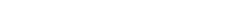The equivalent circuit to create this is:

Here in the example the 2 bits compared by this circuit are in register a and register B

The equivalent code for this comparator in OpenQASM is:

I have created a GATE comparator4 which has the following OPENQASM equivalent code:

gate comparator4 a, b, c, d, f, g, i, j, k, l {
x d;
x j;
x l;
andG1 b, d, f;
andG2 a, b, g;
andG3 a, f, i;
orG1 b, f, j;
x c;
andG4 c, f, k;
x c;
orG2 d, f, l;
swap d, i;
x d;
swap b, g;
}

So we see that this custom quantum gate is made up of a quantum AND gate and a custom quantum OR gate that I created in the following way. If we unfold the doors andG1, andG2, andG3, orG1, orG2 we have:

The OpenQASM equivalent code to write the different gate andG1, andG2, andG3, orG1, orG2 to create comparator 4 is:

gate andG1 a, b, c {
ccx a, b, c;
swap b, c;
}

gate andG2 a, b, c {
ccx a, b, c;
swap b, c;
}

gate andG3 a, b, c {
ccx a, b, c;
swap b, c;
}

gate orG1 a, b, c {
x a;
x b;
ccx a, b, c;
x a;
x b;
swap b, c;
}

gate andG4 a, b, c {
ccx a, b, c;
swap b, c;
}

gate orG2 a, b, c {
x a;
x b;
ccx a, b, c;
x a;
x b;
swap b, c;
}
###### Circuit for checking a sorted list

If we consider here a list of 4 digits stored in the 4 registers of 2 qubits: a, b, m, n. The circuit above is used to test whether the input list is sorted or not. For this I cascaded 3 quantum magnitude comparators (seen in detail in the previous paragraph). Thus the circuit obtained will test if a> b and put the result on the qbit c then check if b> m and store the result on the qbit o and finally it tests if m> n and store the result on the qubit q.

The last quantum gate andOnCompare makes it possible to apply a mutliple AND part: if c AND o AND q are in the quantum state | 1> then the qubit q is set in the quantum state | 1> otherwise it is in the quantum | 0>:

Below is the code of the door andOnCompare in openQASM:

gate andOnCompare a, b, c, d, f {
ccx a, b, d;
swap b, d;
ccx b, c, f;
swap c, f;
}

We have thus created a verification circuit that a list of 4 digits stored in registers a, b, m, n is sorted in a decreasing way or not. The result is stored in the qubit q which has a 100% chance of being measured at state | 1> if the list is sorted otherwise 100% to be at | 0> if the list a, b, m, n is not in decreasing order.

In the general diagram above we notice that in the example:

a=0,b=2,m=3,n=1

in this case this circuit will return the quantum state | 0> on the qubit q

###### Creation of a circuit of permutations

The idea of ​​our algorithm is to permute these 4 digits using control qubits which will be in superimposed state.

The permutation circuit is as follows:

A register of 6 Qubit “control” allows to control the permutation of the list knowing that there are for 4 elements: 4 * 3 * 2 * 1 possible permutations that is to say 24 possibilities the 6 qubits allow to generate these different states.

4 Hadamard quantum gates are used to implement these permutations and take advantage of the superposition of the Qubit ‘control’ register

In other words in the circuit above we see that we start the circuit by initializing the registers a, b, m, n with its list of unsorted numbers, here (a = 0, b = 2, m = 3, n = 1).

The permutation circuit which is controlled by a superimposed qubit register allows the list to be permuted in quantum superposition.

Thus we obtain on the qubit q0 the quantum state | 1> if the permuted list is sorted in a decreasing way otherwise the quantum state | 0>.

The OpenQASM code of the quantum algorithm for performing permutations is as follows:

gate permuter a, b, c, d, f, g, i, j, k, l, m, n, o, p {
cswap k, a, c;
cswap l, a, f;
cswap m, a, i;
cswap k, b, d;
cswap l, b, g;
cswap m, b, j;
cswap n, c, f;
cswap o, c, i;
cswap n, d, g;
cswap o, d, j;
cswap p, f, i;
cswap p, g, j;
}
###### Amplification of the result

I’m going to call this part of my algorithm amplification: the idea is to put the qubit a, b, m, n at | 00>, | 00>, | 00>, | 00> if the list is unsorted. If the list is sorted then the quantum state of a, b, m, n should not be changed.

So we should measure the following result: | 00>, | 00>, | 00>, | 00> when the list is unsorted and a, b, m, n with the input digits in sorted order (in the case in our example this means that there is a probability of measuring the state | 11>, | 10>, | 01>, | 00> either 3,2,1,0 or the sorted list).

The circuit of the quantum amplifier is as follows:

The circuit is based on a series of quantum gate AND applied to the output state q0 AND the number of the list. When the qubit q0 indicates that the list is sorted it is at | 1> and leaves the state of the qubits a, b, m, n at their input state (or permuted by superposition). When the qubit q0 indicates that the list is unsorted it is in the state | 0> the application of the AND gate will thus set the qubits a, b, m, n to | 0>.

The amplifier code is as follows according to a quantum algorithm encoded in OpenQASM:

gate amplifier2 a, b, c, d, f, g, i, j, k, l, m, n, o, p, q, r, v {
ccx a, k, l;
swap a, l;
ccx b, k, m;
swap b, m;
ccx c, k, n;
swap c, n;
ccx d, k, o;
swap d, o;
ccx f, k, p;
swap f, p;
ccx g, k, q;
swap g, q;
ccx i, k, r;
swap i, r;
ccx j, k, v;
swap j, v;
}
###### Complete quantum algorithm for descending sorting of a list of four digits:

Here is the circuit used to sort the following list: a = 0, b = 2, m = 3, n = 1.

It is easily possible to initialize the circuit with another list by modifying the initialization of registers a, b, m, n. Also the current algorithm allows to sort numbers coded on 2 qubits it remains possible to modify the algorithm according to the same principle to sort numbers stored on 3 qubits and consider a list of more than 4 elements (it is then necessary to multiply the number of qubit registers to store the entry list).

The complete code is as follows in openQASM:

OPENQASM 2.0;
include "qelib1.inc";
gate andG1 a, b, c {
ccx a, b, c;
swap b, c;
}

gate andG2 a, b, c {
ccx a, b, c;
swap b, c;
}

gate andG3 a, b, c {
ccx a, b, c;
swap b, c;
}

gate orG1 a, b, c {
x a;
x b;
ccx a, b, c;
x a;
x b;
swap b, c;
}

gate andG4 a, b, c {
ccx a, b, c;
swap b, c;
}

gate orG2 a, b, c {
x a;
x b;
ccx a, b, c;
x a;
x b;
swap b, c;
}

gate comparator a, b, c, d, f, g, i, j, k, l {
x d;
x j;
x l;
andG1 b, d, f;
andG2 a, b, g;
andG3 a, f, i;
orG1 b, f, j;
x c;
andG4 c, f, k;
orG2 d, f, l;
}

gate comparator2 a, b, c, d, f, g, i, j, k, l {
x d;
x j;
x l;
andG1 b, d, f;
andG2 a, b, g;
andG3 a, f, i;
orG1 b, f, j;
x c;
andG4 c, f, k;
orG2 d, f, l;
x d;
swap b, g;
}

gate comparator3 a, b, c, d, f, g, i, j, k, l {
x d;
x j;
x l;
andG1 b, d, f;
andG2 a, b, g;
andG3 a, f, i;
orG1 b, f, j;
x c;
andG4 c, f, k;
orG2 d, f, l;
swap d, i;
x d;
swap b, g;
}

gate comparator4 a, b, c, d, f, g, i, j, k, l {
x d;
x j;
x l;
andG1 b, d, f;
andG2 a, b, g;
andG3 a, f, i;
orG1 b, f, j;
x c;
andG4 c, f, k;
x c;
orG2 d, f, l;
swap d, i;
x d;
swap b, g;
}

gate permutation a, b, c, d, f, g, i, j, k, l, m, n, o, p {
ccx a, c, k;
ccx a, f, l;
ccx a, i, m;
ccx b, d, k;
ccx b, g, l;
ccx b, j, m;
ccx c, f, n;
ccx c, i, o;
ccx d, g, n;
ccx d, j, o;
ccx f, i, p;
ccx g, j, p;
}

gate andOnCompare a, b, c, d, f {
ccx a, b, d;
swap b, d;
ccx b, c, f;
swap c, f;
}

gate permuter a, b, c, d, f, g, i, j, k, l, m, n, o, p {
cswap k, a, c;
cswap l, a, f;
cswap m, a, i;
cswap k, b, d;
cswap l, b, g;
cswap m, b, j;
cswap n, c, f;
cswap o, c, i;
cswap n, d, g;
cswap o, d, j;
cswap p, f, i;
cswap p, g, j;
}

gate amplifier a, b, c, d, f, g, i, j, k, l, m, n, o, p, q, r, v {
ccx l, a, m;
swap a, m;
ccx l, b, n;
swap b, n;
ccx l, c, o;
swap c, o;
ccx l, d, p;
swap d, p;
ccx l, f, q;
swap f, q;
ccx l, f, q;
swap f, q;
ccx l, g, r;
swap g, r;
ccx l, i, v;
swap i, v;
ccx l, j, k;
swap j, k;
}

gate amplifier1 a, b, c, d, f, g, i, j, k, l, m, n, o, p, q, r, v {
ccx a, l, m;
swap a, m;
ccx b, l, n;
swap b, n;
ccx c, l, o;
swap c, o;
ccx d, l, p;
swap d, p;
ccx f, l, q;
swap f, q;
ccx f, l, q;
swap f, q;
ccx g, l, r;
swap g, r;
ccx i, l, v;
swap i, v;
ccx j, k, l;
swap j, k;
}

gate amplifier2 a, b, c, d, f, g, i, j, k, l, m, n, o, p, q, r, v {
ccx a, k, l;
swap a, l;
ccx b, k, m;
swap b, m;
ccx c, k, n;
swap c, n;
ccx d, k, o;
swap d, o;
ccx f, k, p;
swap f, p;
ccx g, k, q;
swap g, q;
ccx i, k, r;
swap i, r;
ccx j, k, v;
swap j, v;
}

qreg a;
qreg b;
qreg m;
qreg n;
qreg c;
qreg o;
qreg q;
qreg d;
qreg g;
qreg j;
qreg k;
qreg l;
qreg r;
qreg uu;
qreg zz;
qreg control;
creg sorted;

reset a;
reset a;
reset b;
reset b;
reset m;
reset m;
reset n;
reset n;
reset c;
reset o;
reset q;
reset d;
reset g;
reset j;
reset k;
reset l;
reset r;
reset uu;
h control;
h control;
h control;
h control;
h control;
h control;
reset a;
reset a;
reset b;
x b;
x m;
x m;
reset n;
reset c;
reset d;
reset g;
reset j;
reset k;
reset l;
x n;
permuter a,a,b,b,m,m,n,n,control,control,control,control,control,control;
comparator4 a,a,b,b,c,d,g,j,k,l;
reset zz;
reset d;
reset g;
reset j;
reset k;
reset l;
comparator4 b,b,m,m,o,d,g,j,k,l;
reset d;
reset g;
reset j;
reset k;
reset l;
comparator4 m,m,n,n,q,d,g,j,k,l;
andOnCompare c,o,q,r,uu;
reset d;
reset g;
reset j;
reset k;
reset l;
reset r;
reset uu;
amplifier2 a,a,b,b,m,m,n,n,q,d,g,j,k,l,r,uu,zz;
measure a -> sorted;
measure a -> sorted;
measure b -> sorted;
measure b -> sorted;
measure m -> sorted;
measure m -> sorted;
measure n -> sorted;
measure n -> sorted;

Here is the Python Qiskit code for my quantum sorting algorithm:

from qiskit import QuantumRegister, ClassicalRegister, QuantumCircuit
from numpy import pi

qreg_a = QuantumRegister(2, 'a')
qreg_b = QuantumRegister(2, 'b')
qreg_m = QuantumRegister(2, 'm')
qreg_n = QuantumRegister(2, 'n')
qreg_c = QuantumRegister(1, 'c')
qreg_o = QuantumRegister(1, 'o')
qreg_q = QuantumRegister(1, 'q')
qreg_d = QuantumRegister(1, 'd')
qreg_g = QuantumRegister(1, 'g')
qreg_j = QuantumRegister(1, 'j')
qreg_k = QuantumRegister(1, 'k')
qreg_l = QuantumRegister(1, 'l')
qreg_r = QuantumRegister(1, 'r')
qreg_uu = QuantumRegister(1, 'uu')
qreg_zz = QuantumRegister(1, 'zz')
qreg_control = QuantumRegister(6, 'control')
creg_sorted = ClassicalRegister(8, 'sorted')
circuit = QuantumCircuit(qreg_a, qreg_b, qreg_m, qreg_n, qreg_c, qreg_o, qreg_q, qreg_d, qreg_g, qreg_j, qreg_k, qreg_l, qreg_r, qreg_uu, qreg_zz, qreg_control, creg_sorted)

circuit.x(qreg_b)
circuit.x(qreg_m)
circuit.x(qreg_m)
circuit.x(qreg_n)
circuit.x(qreg_j)
circuit.x(qreg_l)
circuit.h(qreg_control)
circuit.cswap(qreg_control, qreg_a, qreg_b)
circuit.cswap(qreg_control, qreg_a, qreg_b)
circuit.h(qreg_control)
circuit.cswap(qreg_control, qreg_a, qreg_m)
circuit.cswap(qreg_control, qreg_a, qreg_m)
circuit.h(qreg_control)
circuit.cswap(qreg_control, qreg_a, qreg_n)
circuit.cswap(qreg_control, qreg_a, qreg_n)
circuit.h(qreg_control)
circuit.cswap(qreg_control, qreg_b, qreg_m)
circuit.cswap(qreg_control, qreg_b, qreg_m)
circuit.h(qreg_control)
circuit.cswap(qreg_control, qreg_b, qreg_n)
circuit.x(qreg_b)
circuit.cswap(qreg_control, qreg_b, qreg_n)
circuit.x(qreg_b)
circuit.ccx(qreg_a, qreg_b, qreg_c)
circuit.ccx(qreg_a, qreg_a, qreg_d)
circuit.swap(qreg_a, qreg_d)
circuit.x(qreg_a)
circuit.swap(qreg_b, qreg_c)
circuit.ccx(qreg_a, qreg_c, qreg_g)
circuit.swap(qreg_c, qreg_g)
circuit.x(qreg_c)
circuit.ccx(qreg_a, qreg_c, qreg_j)
circuit.x(qreg_c)
circuit.swap(qreg_c, qreg_j)
circuit.reset(qreg_j)
circuit.x(qreg_j)
circuit.x(qreg_a)
circuit.swap(qreg_a, qreg_d)
circuit.reset(qreg_d)
circuit.ccx(qreg_b, qreg_c, qreg_k)
circuit.swap(qreg_c, qreg_k)
circuit.x(qreg_c)
circuit.reset(qreg_k)
circuit.x(qreg_b)
circuit.x(qreg_b)
circuit.ccx(qreg_b, qreg_c, qreg_l)
circuit.x(qreg_c)
circuit.swap(qreg_c, qreg_l)
circuit.reset(qreg_l)
circuit.x(qreg_l)
circuit.x(qreg_b)
circuit.swap(qreg_b, qreg_g)
circuit.reset(qreg_g)
circuit.x(qreg_b)
circuit.h(qreg_control)
circuit.cswap(qreg_control, qreg_m, qreg_n)
circuit.x(qreg_m)
circuit.x(qreg_n)
circuit.cswap(qreg_control, qreg_m, qreg_n)
circuit.x(qreg_m)
circuit.ccx(qreg_b, qreg_m, qreg_o)
circuit.ccx(qreg_b, qreg_b, qreg_d)
circuit.swap(qreg_b, qreg_d)
circuit.x(qreg_b)
circuit.swap(qreg_m, qreg_o)
circuit.ccx(qreg_b, qreg_o, qreg_g)
circuit.swap(qreg_o, qreg_g)
circuit.x(qreg_o)
circuit.ccx(qreg_b, qreg_o, qreg_j)
circuit.x(qreg_o)
circuit.swap(qreg_o, qreg_j)
circuit.reset(qreg_j)
circuit.x(qreg_j)
circuit.x(qreg_b)
circuit.swap(qreg_b, qreg_d)
circuit.reset(qreg_d)
circuit.ccx(qreg_m, qreg_o, qreg_k)
circuit.swap(qreg_o, qreg_k)
circuit.reset(qreg_k)
circuit.x(qreg_o)
circuit.x(qreg_m)
circuit.x(qreg_m)
circuit.ccx(qreg_m, qreg_o, qreg_l)
circuit.x(qreg_o)
circuit.swap(qreg_o, qreg_l)
circuit.ccx(qreg_c, qreg_o, qreg_r)
circuit.reset(qreg_l)
circuit.x(qreg_l)
circuit.swap(qreg_o, qreg_r)
circuit.reset(qreg_r)
circuit.x(qreg_m)
circuit.swap(qreg_m, qreg_g)
circuit.reset(qreg_g)
circuit.x(qreg_m)
circuit.x(qreg_n)
circuit.ccx(qreg_m, qreg_n, qreg_q)
circuit.ccx(qreg_m, qreg_m, qreg_d)
circuit.swap(qreg_m, qreg_d)
circuit.x(qreg_m)
circuit.swap(qreg_n, qreg_q)
circuit.ccx(qreg_m, qreg_q, qreg_g)
circuit.swap(qreg_q, qreg_g)
circuit.x(qreg_q)
circuit.ccx(qreg_m, qreg_q, qreg_j)
circuit.x(qreg_q)
circuit.swap(qreg_q, qreg_j)
circuit.reset(qreg_j)
circuit.x(qreg_m)
circuit.swap(qreg_m, qreg_d)
circuit.reset(qreg_d)
circuit.ccx(qreg_n, qreg_q, qreg_k)
circuit.swap(qreg_q, qreg_k)
circuit.reset(qreg_k)
circuit.x(qreg_q)
circuit.x(qreg_n)
circuit.x(qreg_n)
circuit.ccx(qreg_n, qreg_q, qreg_l)
circuit.x(qreg_q)
circuit.swap(qreg_q, qreg_l)
circuit.reset(qreg_l)
circuit.ccx(qreg_o, qreg_q, qreg_uu)
circuit.swap(qreg_q, qreg_uu)
circuit.reset(qreg_uu)
circuit.ccx(qreg_a, qreg_q, qreg_d)
circuit.swap(qreg_a, qreg_d)
circuit.x(qreg_n)
circuit.swap(qreg_n, qreg_g)
circuit.reset(qreg_g)
circuit.ccx(qreg_a, qreg_q, qreg_g)
circuit.swap(qreg_a, qreg_g)
circuit.ccx(qreg_b, qreg_q, qreg_j)
circuit.swap(qreg_b, qreg_j)
circuit.ccx(qreg_b, qreg_q, qreg_k)
circuit.swap(qreg_b, qreg_k)
circuit.ccx(qreg_m, qreg_q, qreg_l)
circuit.swap(qreg_m, qreg_l)
circuit.ccx(qreg_m, qreg_q, qreg_r)
circuit.swap(qreg_m, qreg_r)
circuit.ccx(qreg_n, qreg_q, qreg_uu)
circuit.swap(qreg_n, qreg_uu)
circuit.x(qreg_n)
circuit.ccx(qreg_n, qreg_q, qreg_zz)
circuit.swap(qreg_n, qreg_zz)
circuit.measure(qreg_a, creg_sorted)
circuit.measure(qreg_a, creg_sorted)
circuit.measure(qreg_b, creg_sorted)
circuit.measure(qreg_b, creg_sorted)
circuit.measure(qreg_m, creg_sorted)
circuit.measure(qreg_m, creg_sorted)
circuit.measure(qreg_n, creg_sorted)
circuit.measure(qreg_n, creg_sorted)

## Quantum sorting algorithm circuit test with IBM qiskit (python)

The tests are carried out by starting the algorithm on the quantum simulator:

To start the quantum algorithm, you must first initialize:

%matplotlib inline
# Importing standard Qiskit libraries
from qiskit import QuantumCircuit, execute, Aer, IBMQ
from qiskit.compiler import transpile, assemble
from qiskit.tools.jupyter import *
from qiskit.visualization import *
from iqx import *

provider = IBMQ.load_account()

And to perform the test for example by executing the quantum circuit 100 times and displaying the probability of measurement in the form of a histogram:

backend = Aer.get_backend('qasm_simulator')
results = execute(circuit, backend=backend, shots=100).result()
plot_histogram(answer)